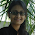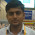# Sql Server Interview Questions and Answers Part 4

1.) Where we generally use Identity column Constraints on a table ?
If any user wants,one column value should  incremented automatically without any  intervention   of users then we generally use this constraints on the column.

2.) How to create a table and add an Identity column on that  table?
Syntax:-
Identity (<seed> < Incremented >)
Example:-
`create table student(eid ``int` `identity(0,1),sname varchar(30), sage ``int``)`
``` ```
3.) How to add Identity  column in Existing table ?
`alter table student add sid ``int` `identity(0,1)`
`                OR`
 `ALTER TABLE student ADD ToBeIdentity INT IDENTITY(0,1)`
``` ```
4.) How to ON/OFF Identity column constraints in sql server using command ?
`set` `identity_insert student on`
`set` `identity_insert student off `
``` ```
`5.)Can we copy data from one table to another table using sql command ?         `
Yes.If Number of column in both table is same.

6.) How to create a new table at run time and copy the data from a existing table using sql command ?

`select *into student2 from student1`
Note:-Here student2  is a new table and student1 is a existing table.

7.) How to copy data in two existing table in sql server using command ?
Syntax:-
insert [first table_name] select *from[second table_name]
Example:-
`insert students select *from studentdetails`

8.) How to copy the data in two existing table if sequence of column name of both table are same ?
Syntax:-
 `insert into [first table_name](column2,column3,column1)select(column2,column3,column1)from [Second table_name]` ``` ``` `Example:-` `insert into student2(sid,sname,sage)select sid, sname,sage from student1`

9.) What are the two sql statement  used to remove the records from database  ?
Syntax:
delete from Table_name where Clause.
Example:
delete from student where sid=101;
Syntax:
truncate table Table_name;
Example:
truncate table student;
9.) What is difference between delete and Truncate statement in sql server ?

• In case of delete each row is deleted one by one and deleted entry is made within log file but In case of truncate table structure is drop and recreated rows are not deleted physically.
• Truncate is faster than delete.
• Within delete statement we can specify 'Where' clause but in truncate statement we can not specify the 'Where' clauses.
• In case of Identity column the difference is,if we run delete statement then insert a new record then identity column value will be the next value of the last inserted values.But if we run truncate statement and insert a new record the next value seed value.
• In case of Foreign key constraint the difference is, if child table does not have any record then we can run delete statement against parents table but we can not run truncate statement against parent table.
• Delete is DML Statement and truncate is DDL Statement.
• If record is deleted that can be recover but if record is truncated then can not recover.

1.1.